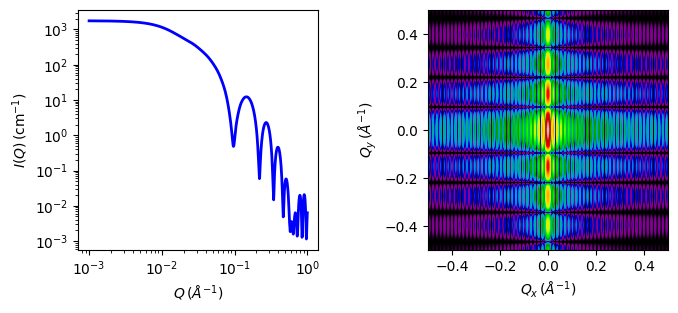# hollow_cylinder

Parameter

Description

Units

Default value

scale

Scale factor or Volume fraction

None

1

background

Source background

cm-1

0.001

20

thickness

Cylinder wall thickness

10

length

Cylinder total length

400

sld

Cylinder sld

10-6-2

6.3

sld_solvent

Solvent sld

10-6-2

1

theta

Cylinder axis to beam angle

degree

90

phi

degree

0

The returned value is scaled to units of cm-1 sr-1, absolute scale.

Definition

This model provides the form factor, $$P(q)$$, for a monodisperse hollow right angle circular cylinder (rigid tube) where the The inside and outside of the hollow cylinder are assumed to have the same SLD and the form factor is thus normalized by the volume of the tube (i.e. not by the total cylinder volume).

$P(q) = \text{scale} \left<F^2\right>/V_\text{shell} + \text{background}$

where the averaging $$\left<\ldots\right>$$ is applied only for the 1D calculation. If Intensity is given on an absolute scale, the scale factor here is the volume fraction of the shell. This differs from the core_shell_cylinder in that, in that case, scale is the volume fraction of the entire cylinder (core+shell). The application might be for a bilayer which wraps into a hollow tube and the volume fraction of material is all in the shell, whereas the core_shell_cylinder model might be used for a cylindrical micelle where the tails in the core have a different SLD than the headgroups (in the shell) and the volume fraction of material comes fromm the whole cyclinder. NOTE: the hollow_cylinder represents a tube whereas the core_shell_cylinder includes a shell layer covering the ends (end caps) as well.

The 1D scattering intensity is calculated in the following way (Guinier, 1955)

$\begin{split}P(q) &= (\text{scale})V_\text{shell}\Delta\rho^2 \int_0^{1}\Psi^2 \left[q_z, R_\text{outer}(1-x^2)^{1/2}, R_\text{core}(1-x^2)^{1/2}\right] \left[\frac{\sin(qHx)}{qHx}\right]^2 dx \\ \Psi[q,y,z] &= \frac{1}{1-\gamma^2} \left[ \Lambda(qy) - \gamma^2\Lambda(qz) \right] \\ \Lambda(a) &= 2 J_1(a) / a \\ \gamma &= R_\text{core} / R_\text{outer} \\ V_\text{shell} &= \pi \left(R_\text{outer}^2 - R_\text{core}^2 \right)L \\ J_1(x) &= (\sin(x)-x\cdot \cos(x)) / x^2\end{split}$

where scale is a scale factor, $$H = L/2$$ and $$J_1$$ is the 1st order Bessel function.

NB: The 2nd virial coefficient of the cylinder is calculated based on the outer radius and full length, which give an the effective radius for structure factor $$S(q)$$ when $$P(q) \cdot S(q)$$ is applied.

In the parameters,the radius is $$R_\text{core}$$ while thickness is $$R_\text{outer} - R_\text{core}$$.

To provide easy access to the orientation of the core-shell cylinder, we define the axis of the cylinder using two angles $$\theta$$ and $$\phi$$ (see cylinder model).Fig. 31 1D and 2D plots corresponding to the default parameters of the model.

Source

hollow_cylinder.py $$\ \star\$$ hollow_cylinder.c $$\ \star\$$ gauss76.c $$\ \star\$$ sas_J1.c $$\ \star\$$ polevl.c

References

1. L A Feigin and D I Svergun, Structure Analysis by Small-Angle X-Ray and Neutron Scattering, Plenum Press, New York, (1987)

1. Onsager, Ann. New York Acad. Sci., 51 (1949) 627-659

Authorship and Verification

• Author: NIST IGOR/DANSE Date: pre 2010